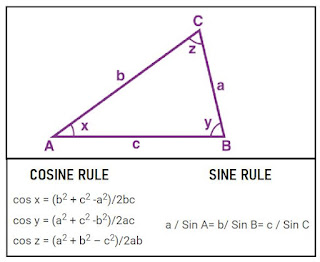# Difference between Triangulation & Trilateration in Surveying

Triangulation and trilateration are two methods used to conduct land surveying by determining horizontal measurements. The basic difference between triangulation and trilateration is that, triangulation involves measurement of angles in surveying, while trilateration involves measurement of length. The process involves measurement of sides or angles of an area that is divided into triangles, polygons, quadrilateral or a combination of figures.

No Time To Read?? Watch Video: Here

 TRIANGULATION TRILATERATION The triangles formed in the area is determined by measuring their angles. The triangles formed in the area is determined by measuring their sides. A base line whose value is known is taken as a reference for proceeding the measurement. The azimuth of a line is the known value and it is taken as the reference to proceed the measurement. To control scale error, check base lines are measured. To control angular error, check angles are measured Intervisibility between stations is essential It is possible to measure distances without intervisibility More internal checks Less internal checks compared to triangulation Once the angles are measured, the sides are sides of the triangles are calculated using sine rule.In the above figure, if the anyone side for example baseline ,AB and the angles A, B and C are known, then as per sine rule,BC/sin A = AC/ sin B = AB/ sin C; Once the sides of the triangular figures are determined, the angles are calculated using cosine law.Now, if the sides of the triangle are known, the angles are determined using cosine rule.Read More Articles On Surveying>>> Here